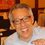# Corollary to Euler's Theorem

When we studied Euler's Theorem back in college (in Switzerland), the professor mentioned the following simple corollary, leaving the proof as an exercise: $a^{\phi(n)+k}\equiv{a^k}\pmod{n}$ for all positive integers $a$ and $n$, as long as $k$ is $\geq$ the multiplicity of all the primes in the factorization of $n$. Can you prove (or disprove) this?

For example, if $n=2^3\times{5}\times{7^4}$ , we want $k\geq{4}$

Thus we can say that "in modular arithmetic, all exponential functions eventually become periodic."Note by Otto Bretscher
6 years, 2 months ago

This discussion board is a place to discuss our Daily Challenges and the math and science related to those challenges. Explanations are more than just a solution — they should explain the steps and thinking strategies that you used to obtain the solution. Comments should further the discussion of math and science.

When posting on Brilliant:

• Use the emojis to react to an explanation, whether you're congratulating a job well done , or just really confused .
• Ask specific questions about the challenge or the steps in somebody's explanation. Well-posed questions can add a lot to the discussion, but posting "I don't understand!" doesn't help anyone.
• Try to contribute something new to the discussion, whether it is an extension, generalization or other idea related to the challenge.

MarkdownAppears as
*italics* or _italics_ italics
**bold** or __bold__ bold
- bulleted- list
• bulleted
• list
1. numbered2. list
1. numbered
2. list
Note: you must add a full line of space before and after lists for them to show up correctly
paragraph 1paragraph 2

paragraph 1

paragraph 2

[example link](https://brilliant.org)example link
> This is a quote
This is a quote
    # I indented these lines
# 4 spaces, and now they show
# up as a code block.

print "hello world"
# I indented these lines
# 4 spaces, and now they show
# up as a code block.

print "hello world"
MathAppears as
Remember to wrap math in $$ ... $$ or $ ... $ to ensure proper formatting.
2 \times 3 $2 \times 3$
2^{34} $2^{34}$
a_{i-1} $a_{i-1}$
\frac{2}{3} $\frac{2}{3}$
\sqrt{2} $\sqrt{2}$
\sum_{i=1}^3 $\sum_{i=1}^3$
\sin \theta $\sin \theta$
\boxed{123} $\boxed{123}$

Sort by:

Let me try to outline a proof.

It suffices to prove the congruency modulo all the prime power factors of $n$. For example, if $n=2^3\times{5}\times{7^4}$ , it suffices to prove the congruency modulo $2^3, 5$ and $7^4$.

So, let $p^m$ be a prime power factor of $n$. If $p\not|{a}$, then we have $a^{\phi(p^m)}\equiv{1}\pmod{p^m}$ by Euler's Theorem. Since $\phi(p^m)|\phi(n)$ , we have $a^{\phi(n)}\equiv{1}\pmod{p^m}$ as well and therefore $a^{\phi(n)+k}\equiv{a^k}\pmod{p^m}$ as claimed.

If $p|a$, then $p^m|a^k$ since $k\geq{m}$ , so that $a^{\phi(n)+k}\equiv{0}\equiv{a^k}\pmod{p^m}.$

- 6 years, 2 months ago

Can't we use euler's theorem and multiply a^k to both sides giving result.

- 6 years, 2 months ago

Euler's theorem is valid iff $\gcd(a,n)=1$ where $a,n\in\Bbb{Z^+}$. The result in this note, however, doesn't have the restriction that $\gcd(a,n)=1$ and hence you cannot directly use Euler's Theorem here.

- 6 years, 2 months ago

Forgot it .Now it seems slight difficult thanx.

- 6 years, 2 months ago

Ohk , thanx!

- 6 years, 2 months ago

Ya here we have to deal extra case of gcd not being 1 rest seems using euler theorem and some arithmetic .

- 6 years, 2 months ago

Yes i also thought the same.....

- 6 years, 2 months ago

@Tijmen Veltman proved this for $k=1$ and square-free $n$ in this note.

He might be interested in proving/disproving this one too, so I'm tagging him here.

- 6 years, 2 months ago

Interesting! Thank you for letting me know.

The result I quote is sometimes mentioned in introductory Number Theory texts, usually as an exercise. I have never found any use for it... until I joined Brilliant ;)

- 6 years, 2 months ago

Let the GCD of $a$ and $n$ be $g$. Then, $a=gc$ and $n=gd$.

The rest of the proof is left to the reader as an exercise. (Just kidding, I got to go now and I will finish it later.)

- 5 years, 9 months ago

I made a problem about this a while back (although I just now read this note): Divisibility of power differences. It was when I was writing the wiki on Carmichael numbers.

- 5 years, 2 months ago

Proof for the corollary:

let $n = p_{1}^{a_{1}}p_{2}^{a_{2}}...p_{m}^{a_m}$be the standard factorisation of $n$.

$k$ is greater than the multiplicity of all the primes in the factorization of $n$ is equivalent to $k \geq max\left\{a_1, a_2, ..., a_m\right\}$.

if $a, p_i$ are co-prime, $a^{\phi(n) + k} = a^{\phi(p_{1}^{a_{1}})\phi(p_{2}^{a_{2}})...\phi(p_{m}^{a_m}) + k} \equiv a^k \pmod{p_{i}^{a_i}}$.

if $a, p_i$ are not co-prime, $v_{p_i}(a^k) = kv_{p_i}(a) \geq a_i \Rightarrow a^k \equiv 0 \pmod{p_{i}^{a_i}}$, where $v_{p}(n)$ denotes the exponent of $p$ in the prime factorization of $n$. $\phi(n) + k > k \Rightarrow a^{\phi(n) + k} \equiv 0 \pmod{p_{i}^{a_i}}$. Hence $a^{\phi(n) + k} \equiv a^k \equiv 0 \pmod{p_{i}^{a_i}}$.

Hence, for all positive $a, n$, $a^{\phi(n) + k} \equiv a^k \pmod{p_{i}^{a_i}}$.

Since this is true for all $i \in \left\{1, 2, ..., m\right\}$, the result follows.

- 1 year, 4 months ago